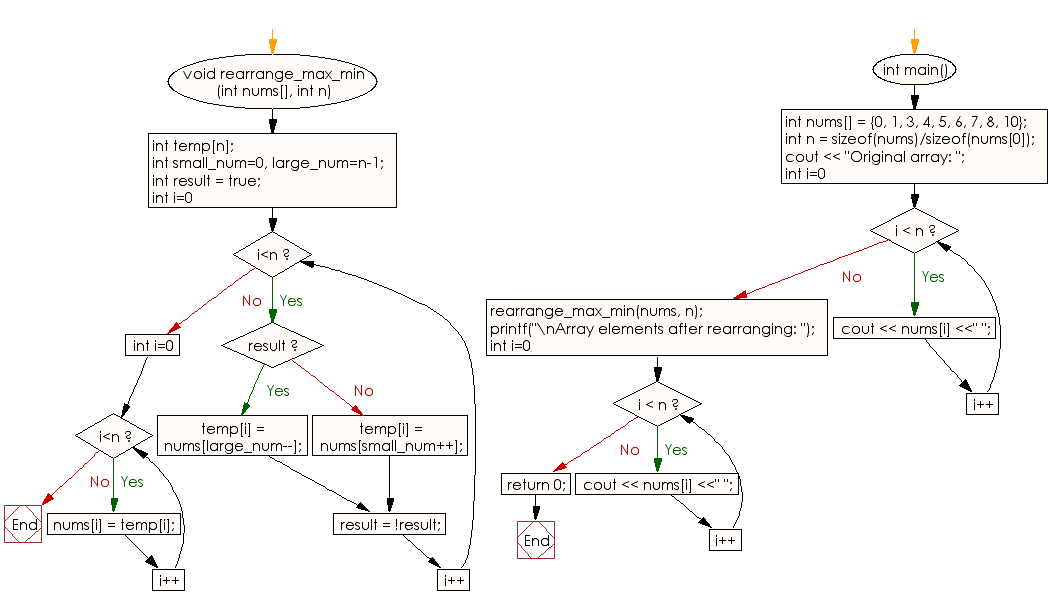﻿ C++ : Rearrange a given sorted array of positive integers# C++ Exercises: Rearrange a given sorted array of positive integers

## C++ Array: Exercise-15 with Solution

Write a C++ program to rearrange a given sorted array of positive integers.
Note: In final array, first element should be maximum value, second minimum value, third second maximum value, fourth second minimum value, fifth third maximum and so on.

Sample Solution:

C++ Code :

``````#include <iostream>
using namespace std;

void rearrange_max_min(int nums[], int n)
{
int temp[n];
int small_num=0, large_num=n-1;
int result = true;

for (int i=0; i<n; i++)
{
if (result)
temp[i] = nums[large_num--];
else
temp[i] = nums[small_num++];

result = !result;
}

for (int i=0; i<n; i++)
nums[i] = temp[i];
}

int main()
{
int nums[] = {0, 1, 3, 4, 5, 6, 7, 8, 10};
int n = sizeof(nums)/sizeof(nums);
cout << "Original array: ";
for (int i=0; i < n; i++)
cout << nums[i] <<" ";
rearrange_max_min(nums, n);

printf("\nArray elements after rearranging: ");
for (int i=0; i < n; i++)
cout << nums[i] <<" ";
return 0;

}
``````

Sample Output:

```Original array: 0 1 3 4 5 6 7 8 10
Array elements after rearranging: 10 0 8 1 7 3 6 4 5
```

Flowchart:C++ Code Editor:

What is the difficulty level of this exercise?

Test your Programming skills with w3resource's quiz.

﻿